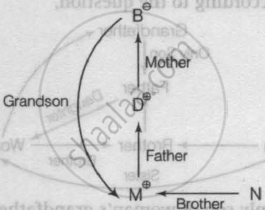# The Following Question is Based on the Information Given Below. 'P X Q' Means 'P is the Father of Q' 'P - Q' Means 'P is the Sister of Q'. 'P + Q' Means 'P is the Mother of Q'. 'P +Q' Means 'Pis Brot - Logical Reasoning

MCQ

The following question is based on the information given below.

1. 'P x Q' means 'P is the father of Q'
2. 'P - Q' means 'P is the sister of Q'.
3. 'P + Q' means 'P is the mother of Q'.
4. 'P +Q' means 'P is the brother of Q'

In the expression B + D x M ÷ N, M related to B?

#### Options

• Granddaughter

• Son

• Grandson

• Granddaughter or Grandson

#### Solution

Grandson
Explanation:
According to the information,
B + D x M ÷ NSo, M is the grandson of B.

Concept: Blood Relationships (Entrance Exam)
Is there an error in this question or solution?